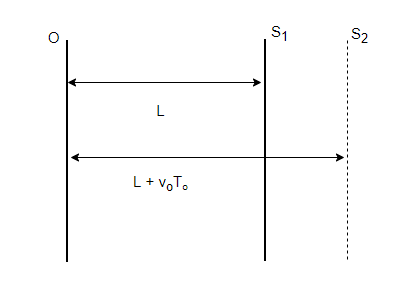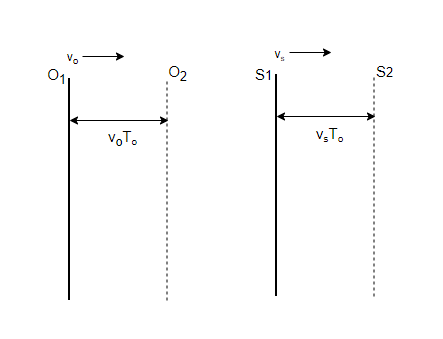GFG App
Open AppBrowser
Continue

# Doppler Effect – Definition, Formula, Examples

Doppler Effect is an important phenomenon when it comes to waves. This phenomenon has applications in a lot of fields of science. From nature’s physical process to planetary motion, this effect comes into play wherever there are waves and the objects are traveling with respect to the wave. In the real world surrounding us, it can be seen when vehicles such as an ambulance are traveling near a person. The person then observes that the frequency of the siren of the ambulance changes when the ambulance approaches and then goes past that person. With so many instances of play of this effect, it becomes essential to study this effect. Let’s look at this effect in detail.

## Doppler Effect

In an everyday experience as discussed above, the frequency of the whistle of a train changes as the train approaches the station and then moves away from it. When someone approaches a stationary source of sound with speed. The pitch of the sound from the source appears to be more than its actual pitch. As the observer goes away from the source, the observed frequency seems to be lower than the actual frequency of the sound from the source. This motion-related change in observed frequency is called Doppler Effect

It is a wave phenomenon, it doesn’t hold only for sound waves. It holds for all the waves. There are three possible cases that should be analyzed while studying the Doppler effect:

1. The observer is stationary but the source is moving.
2. The observer is moving but the source is stationary.
3. Both source and observer are moving.

Note: Most waves require a medium for propagation, however electromagnetic waves do not require any medium. In that case, these case of observer moving and source stationary and vice-versa are same.

Source Moving: Observer stationery

A train approaching a station is an example of a sound source approaching its observer. The figure given below shows a situation where the source is moving and the object is stationary. The velocity of the object is denoted by vs. If the actual frequency of the source is denoted by f and f’ denotes the observed frequency. If “v” denotes the velocity of the sound wave. Then the observed frequency, in this case, is given by,Source stationary: Observer Moving

An observer sitting in the car is approaching the stadium where a concert is happening. This is an example of a situation where the observer moves and approaches the source. In a situation where the source is stationary and the object is moving. The velocity of the object is denoted by vo. If the actual frequency of the source is denoted by f and f’ denotes the observed frequency. If “v” denotes the velocity of the sound wave. Then the observed frequency, in this case, is given by,Both Source and Observer Moving

The figure is given below which shows the scenario where both source and observer were moving. The velocity of the object is denoted by vo while the velocity of the source is given by vs. If the actual frequency of the source is denoted by f and f’ denotes the observed frequency. If “v” denotes the velocity of the sound wave. Then the observed frequency, in this case, is given by,Notice that the third case combines both of the previous two cases. This means that the third case is a generalization of both the previous cases. In case the direction of velocity is reversed. The signs of the velocity are changed accordingly.

Limitations of Doppler Effect

Even though the doppler effect has applications in almost every field where the waves are present, it still has certain limitations. It is only applicable in the situations where velocities of sound and velocities of objects are much less than the speed of sound in that medium. Also, in the case of Doppler effect formulation, the motion of the object and the source must be along the same lines.

Applications of Doppler Effect

1. Used in estimating the speed of distant stars, planets, and other celestial bodies.
2. Used in finding the velocities of airplanes and submarines.
3. In police radar systems, it is used to measure the speed of automobiles.

## Sample Problems

Question 1: An ambulance is approaching a person at a speed of 3 m/s. Assuming that the frequency of the siren of the ambulance is 440Hz. Find out the frequency at which the observer hears the siren. (Velocity of the sound in air = 360 m/s).

In this case, the source is moving towards the observer.Given: f = 440Hz, V = 360 m/s and Vs = 3 m/s

Plugging the values in the equation,⇒⇒⇒⇒ f’ = 443 Hz .

Question 2: An ambulance is receding away from a person at a speed of 6 m/s. Assuming that the frequency of the siren of the ambulance is 440Hz. Find out the frequency at which the observer hears the siren. (Velocity of the sound in air = 360 m/s).

In this case, the source is moving towards the observer.Given: f = 440Hz, V = 360 m/s and Vs = 6 m/s

Plugging the values in the equation,⇒⇒⇒ f’ = 432.7 Hz .

Question 3: An person is approaching a stadium on a motorcycle at a speech of 10m/s. Assuming that the frequency of the sound coming out of the stadium is 400Hz. Find out the frequency which the person hears in the sound. (Velocity of the sound in air = 360 m/s).

In this case, the observer is moving towards the source.Given: f = 400Hz, V = 360 m/s and Vo =10 m/s

Plugging the values in the equation,⇒⇒⇒ f’ = 411 Hz .

Question 4: An person is approaching a van moving at a speed of 20 m/s on a motorcycle at a speech of 10m/s. Assuming that the frequency of the sound of the horn coming out from the van is 400Hz. Find out the frequency which the person hears in the sound. (Velocity of the sound in air = 360 m/s).

In this case, both the observer and source are moving. .Given: f = 400Hz, V = 360 m/s, Vo =10 m/s and vs  = 20m/s

Plugging the values in the equation,⇒⇒⇒ f’ = 434 Hz.

Question 5: An person is approaching a van moving away at a speed of 20 m/s on a motorcycle at a speech of 10m/s. Assuming that the frequency of the sound of the horn coming out from the van is 400Hz. Find out the frequency which the person hears in the sound. (Velocity of the sound in air = 360 m/s).

In this case, both the observer and source are moving. .Given: f = 400Hz, V = 360 m/s, Vo =10 m/s and vs  = 20m/s

Plugging the values in the equation,⇒⇒⇒ f’ = 367 Hz.

My Personal Notes arrow_drop_up